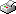Print This Page Ellinor Fackle Fornius -- doctoral thesis -- Optimal Design of Experiments for the Quadratic Logistic Model Abstract Optimal design of experiments for binary data is the topic of this thesis. A particular logistic model including a quadratic term in the linear predictor is considered. Determining an optimal design for this model is complicated by the fact that the optimal design is dependent on the unknown true parameters. Methods to obtain locally c- and D-optimal designs are illustrated. c-optimal designs are derived via the canonical design space. This space offers an useful geometric interpretation of the design problem. Using the canonical design space it is shown how the number of design points in a c-optimal design varies depending on the parameter being estimated. Furthermore, formulae for finding the design points along with the corresponding design weights are derived. The small sample performance of the locally optimal designs is compared to the performances of some non-optimal designs in a simulation study. The evaluations are made in terms of mean squared error of the maximum likelihood estimator. The small sample distribution of the maximum likelihood estimator is demonstrated to be quite different from the asymptotic distribution. It was also concluded that non-existence of the maximum likelihood estimator is a critical problem for the quadratic logistic model. The designs differed considerably in this respect and this problem also turned out to be parameter dependent. As a solution to this problem another type of parameter estimator is suggested, which is also evaluated in the simulation study. It performs better in this respect, but not completely satisfactory because it fails in other respects. Two kinds of sequential design approaches are proposed for the purpose of finding the point of optimum response. One is a parametric optimal design approach where c-optimal designs are updated sequentially. The other one is a nonparametric stochastic approximation approach. The suggested designs are evaluated and compared via simulations. Based on the simulation results the c-optimal design approach was consistently favored. Sequential estimation proved to be an effective way to handle the parameter dependence issue. Keywords: Logistic regression, D-optimality, c-optimality, Canonical design space, Maximum likelihood estimation, Sequential design, Stochastic approximation. ISBN 978-91-7155-644-8